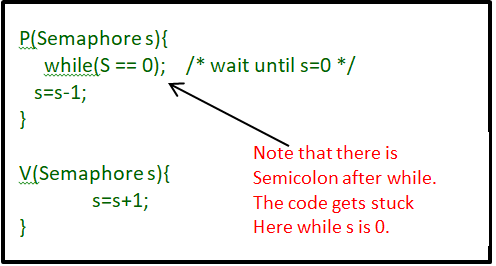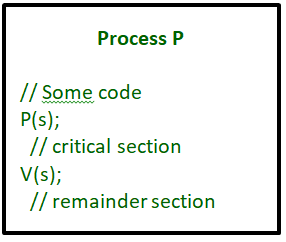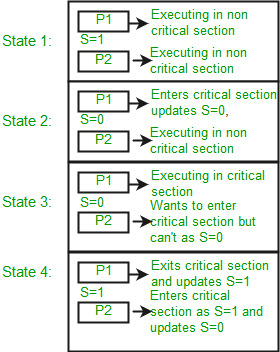Operating System | Semaphores in operating system

Prerequisite: process-synchronization, Mutex vs Semaphore

Semaphore is simply a variable. This variable is used to solve the critical section problem and to achieve process synchronization in the multiprocessing environment.
The two most common kinds of semaphores are counting semaphores and binary semaphores. Counting semaphore can take non-negative integer values and Binary semaphore can take the value 0 & 1. only.

Now let us see how it do so.

First, look at two operations which can be used to access and change the value of the semaphore variable.Some point regarding P and V operation

1. P operation is also called wait, sleep or down operation and V operation is also called signal, wake-up or up operation.
2. Both operations are atomic and semaphore(s) is always initialized to one.
3. A critical section is surrounded by both operations to implement process synchronization.See below image.critical section of Process P is in between P and V operation.Now, let us see how it implements mutual exclusion. Let there be two processes P1 and P2 and a semaphore s is initialized as 1. Now if suppose P1 enters in its critical section then the value of semaphore s becomes 0. Now if P2 wants to enter its critical section then it will wait until s > 0, this can only happen when P1 finishes its critical section and calls V operation on semaphore s. This way mutual exclusion is achieved. Look at the below image for details.The description above is for binary semaphore which can take only two values 0 and 1. There is one other type of semaphore called counting semaphore which can take values greater than one.

Now suppose there is a resource whose number of instance is 4. Now we initialize S = 4 and rest is same as for binary semaphore. Whenever process wants that resource it calls P or wait function and when it is done it calls V or signal function. If the value of S becomes zero then a process has to wait until S becomes positive. For example, Suppose there are 4 process P1, P2, P3, P4 and they all call wait operation on S(initialized with 4). If another process P5 wants the resource then it should wait until one of the four processes calls signal function and value of semaphore becomes positive.

Problem in this implementation of semaphore

Whenever any process waits then it continuously checks for semaphore value (look at this line while (s==0); in P operation) and waste CPU cycle. To avoid this another implementation is provided below.

 `P(Semaphore s) ` `{ ` `    ``s = s - 1; ` `    ``if` `(s <= 0) { ` ` `  `        ``// add process to queue ` `        ``block(); ` `    ``} ` `} ` ` `  `V(Semaphore s) ` `{ ` `    ``s = s + 1; ` `    ``if` `(s <= 0) { ` ` `  `        ``// remove process p from queue ` `        ``wakeup(p); ` `    ``} ` `} `

In this implementation whenever process waits it is added to a waiting queue of processes associated with that semaphore. This is done through system call block() on that process. When a process is completed it calls signal function and one process in the queue is resumed. It uses wakeup() system call.

This article is contributed by Ashish Sharma. If you like GeeksforGeeks and would like to contribute, you can also write an article using contribute.geeksforgeeks.org or mail your article to contribute@geeksforgeeks.org. See your article appearing on the GeeksforGeeks main page and help other Geeks.

My Personal Notes arrow_drop_up

Article Tags :
Practice Tags :

20

Please write to us at contribute@geeksforgeeks.org to report any issue with the above content.